# ISEE Lower Level Quantitative : Data Analysis

## Example Questions

← Previous 1 3 4 5 6

### Example Question #1 : How To Identify The Parts Of A List

The players on Rebecca's basketball team are the following ages: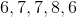What is the median age?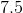Explanation:

In order to find the median, the numbers in a set must be ordered from smallest to largest, and the middle number identified. Doing this results in: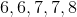The middle number is 7, which is therefore the median.

### Example Question #2 : How To Identify The Parts Of A List

Greg is a shoe salesman. The past 5 days, he has sold the following numbers of shoes: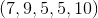What is the median?Explanation:

The median is the middle number in a set when ordered from smallest to largest.

When the set,is ordered from smallest to largest, it is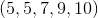Here, the middle number is 7. Therefore, the correct answer is 7.

### Example Question #3 : How To Identify The Parts Of A List

What is the mode of the set below?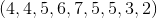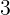Explanation:

The mode of a set is the number that appears most often. Given that the number 5 appears 3 times (more than any other number) it is therefore the mode and the correct answer.

### Example Question #4 : How To Identify The Parts Of A List

If Gene has 4 dogs that are 8 years old, 5 years old, 7 years old, and 2 years old, what is the average age?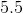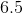Explanation:

The average of a set is the sum of the numbers in the set divided by the number of items in the set.

Therefore, the average is equal to: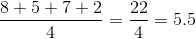### Example Question #5 : How To Identify The Parts Of A List

If Angela runs 5 miles on Tuesday, 6 miles on Wednesday, and 7 miles on Thursday, how many miles will she run on Saturday if she continues with this pattern?Explanation:

Angela's pattern is to run one more mile each subsequent day than the number of miles she ran the previous day. Therefore, since she ran 7 miles on Thursday, she will run 8 miles on Friday and 9 miles on Saturday. Therefore, 9 is the correct answer.

### Example Question #6 : How To Identify The Parts Of A List

Which of the following could not be a term in the sequence 5, 10, 15, 20...?

10005

3751

35

2500

3751

Explanation:

All answers in the sequence must end in a 5 or a 0.

### Example Question #7 : How To Identify The Parts Of A List

Throughout the lastgames of the basketball season,  Joleen scored the following number of points: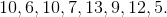What is the average amount of points that Joleen scored per game?Explanation:

To find the average number of points that Joleen scored, first find the sum of Joleen's points. Then divide the sum by the number of games to determine the average per game. Thus, the solution is: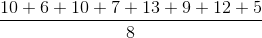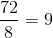### Example Question #8 : How To Identify The Parts Of A List

What is the range of the following set of numbers: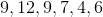Explanation:

To calculate the range of the set, find the difference between the greatest and lowest numbers. Thus, the solution is: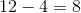### Example Question #1 : How To Identify The Parts Of A List

The following set of numbers is the final exam scores from Ms. Turk's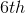grade English exam. Find the median score.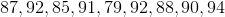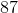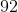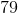Explanation:

To find the median exam score, arrange the list in descending or ascending order. The median is the middle score. Thus, the solution is: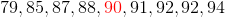### Example Question #10 : How To Identify The Parts Of A List

The following sequence of numbers is the amount of hours that Carlos spent playing video games during each of the pastweeks. Find the mean number of hours that Carlos spent playing video games per week.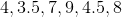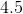Explanation:

To find the mean number of hours that Carlos played per week, calculate the sum total of all of the hours that he played video games during the past six weeks. Then divide the sum by the total number of weeks.

Thus, the solution is: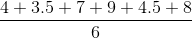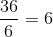← Previous 1 3 4 5 6